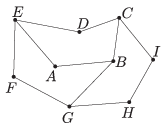Mathematical and Physical Journal
for High Schools
Issued by the MATFUND Foundation
 Already signed up? New to KöMaL?

#Problem C. 1240. (September 2014)

C. 1240. Pentagon $\displaystyle ABCDE$ is obtained by rotating pentagon $\displaystyle HICBG$ about $\displaystyle C$, and pentagon $\displaystyle FGBAE$ is obtained by rotating pentagon $\displaystyle ABCDE$ about $\displaystyle E$, as shown in the sketch. The length of line segment $\displaystyle AB$ is 7 cm.What is the total length of the 11 line segmets?

Based on a German competition problem

(5 pont)

Deadline expired on October 10, 2014.

### Statistics:

 372 students sent a solution. 5 points: 311 students. 4 points: 28 students. 3 points: 11 students. 1 point: 3 students. 0 point: 10 students. Unfair, not evaluated: 9 solutionss.

Problems in Mathematics of KöMaL, September 2014